# Tile Patterns II: hexagons

Alignments to Content Standards: 8.G.A.5

A common tiling pattern with hexagons is pictured below:1. A regular hexagon is a hexagon with $6$ congruent sides and $6$ congruent interior angles. Find the measure of the interior angles in a regular hexagon.
2. Show that three equally sized regular hexagons sharing a common vertex can be arranged in the configuration of the above picture.
3. Show that a single regular hexagon can be surrounding by six regular hexagons with no spaces and no overlap, as in the picture below: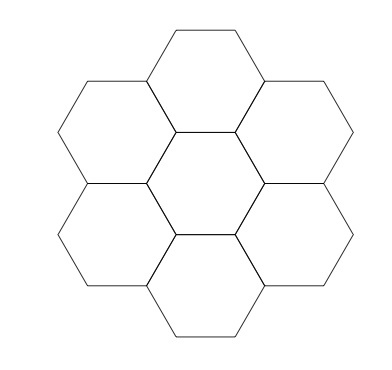## IM Commentary

Tile patterns will be familiar with students both from working with geometry tiles and from the many tiles they encounter in the world. Here one of the most important examples of a tiling, with regular hexagons, is studied in detail. This provides students an opportunity to use what they know about the sum of the angles in a triangle and also the sum of angles which make a line. If hexagonal and equilateral triangular tiles are available, the teacher may wish to use them to help students visualize.

The pattern of equally sized regular hexagons fitting together to fill the plane is familiar not only from tile but also in nature:

• the molecular structure of graphene (a one atom thin ''sheet'' of graphite) consists of carbon atoms placed at the vertices of regular hexagons,
• convection patterns for thin layers of heated liquids,
• beehives are often built in this pattern of hexagons.

This task is ideally suited for instruction purposes where students can take their time and develop several of the Mathematical Practice standards, as the mathematical content is directly related to, but somewhat exceeds, the content of standard 8.G.5 on sums of angles in triangles. Careful analysis of the angles requires students to construct valid arguments (MP3) using abstract and quantitative reasoning (MP2). Producing the picture in part (c) helps students identify a common mathematical argument repeated multiple times (MP8). If students use pattern blocks in order to develop the intuition for decomposing the hexagon into triangles, then this is also an example of MP5.

## Solutions

Solution: Measuring angles

1. One way to calculate the measure of the interior angles on the hexagon is the following. Suppose you are travelling counterclockwise along the hexagon. At each corner, you make a turn. After $6$ of these turns you are heading in the same direction in which you started and have made one full revolution of $360^\circ$. Since the angles in the regular hexagon are all congruent, each of these turns must be $\frac{360^\circ}{6} = 60^\circ$. Since the sum of the interior angle and $60^\circ$ is $180^\circ$, this means that the interior angle must measure $120^\circ$: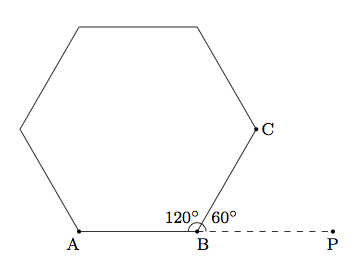In the picture, the interior angle is angle $ABC$ while the $60^\circ$ ''turn'' is angle $PBC$.

2. The picture below includes a second hexagon adjacent to the first: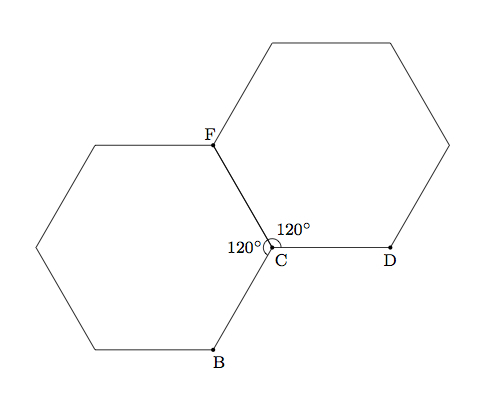We know that $$m(\angle BCD) + m(\angle BCF) + m(\angle DCF) = 360.$$ Since $m(\angle BCF) = m(\angle DCF) = 120$ from part (a) we conclude that $m(\angle BCD) = 120$. This means, from part (a), that angle $BCD$ forms an interior angle of a regular hexagon congruent to the two hexagons pictured in part (a). In other words, a third hexagon can be placed as in the picture: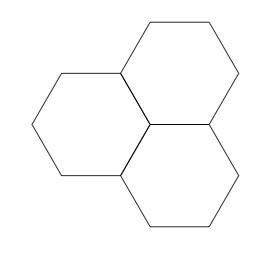3. The method of part (b) needs to be applied four more times, inserting a new hexagon in each open space, with one side coming from the central hexagon and one side coming from one of the adjacent hexagons. Continuing to work around clockwise, the hexagons below are labelled in the order in which they are added to the initial hexagon: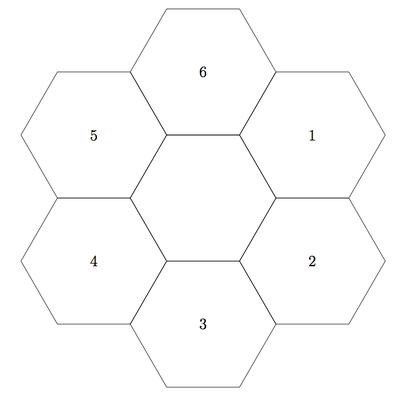At the last step, we have three sides of the sixth hexagon already in place: they are all congruent and form two $120^\circ$ angles so the sixth hexagon will fit in place to complete the set of seven hexagons.

Solution: 2 Triangulating Hexagon (alternate solution for part a)

We can divide the hexagon into triangles by adding a vertex at the center of the hexagon as pictured below: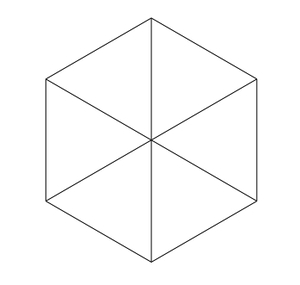Since there are $360^\circ$ in a circle, each of the six interior angles for the triangles measures $60^\circ$. The six triangles are isosceles since the vertex is at the center of the regular hexagon. This means that the base angles of the six triangles also each measure $60^\circ$. So these six triangles are equilateral. Each interior angle of the hexagon is made up of two angles of equilateral triangles and so they must each measure $120^\circ$.